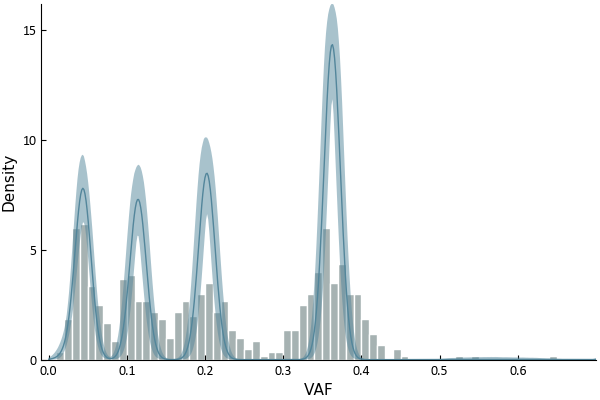dummy-link

5

0

1

1

# DPClustering

Perform Dirichlet clustering on Varaint Allele Frequncies (VAFs) from sequencing data of cancers a la Nik-Zainal et al.

## Getting started

Package is written in the Julia programming language.

To download this package use the `Pkg.add` function as below, which will download the package and install all the dependencies.

``````Pkg.add("DPClustering")
``````

## Clustering

Clustering is invoked using the `dpclustering` function which takes 2 vectors of equal size: y - the number of reads reporting the mutation and N - the depth of coverage at that locus. With these, clustering can be performed and the function will return a `DPresults` type. There are a number of optional arguments which are all set to reasonable defaults, you may want to change the number of iterations or set verbose to false. the default is to show a log of the time taken in the gibbs sampler. To see the optional arguments and their defaults use `?dpclustering` in a julia session.

``````dp = dpclustering(y, N, iterations = 10000, verbose = false);
``````

You can then plot the output using `plotresults(dp)`.

At the moment, clustering will only work with single samples and mutations in copy neutral regions. So the input mutations should either be filtered for copy number alterations or corrected for copy number before inputting.

## Example

There is some example data provided originally in Nik-Zainal et al in the examples folder. So an analysis would proceed as follows. There is a built in function to plot the data and associated clustering.

``````using DPClustering
data = readcsv("example/data.csv", header = true)
y = data[:, 1]
N = data[:, 2]

out = dpclustering(y, N)
myplot = plotresults(out, save = true)
``````## Speed

Due to Julia's just in time compilation the sampling is relatively fast. For example, the analysis above (600 mutations) the time taken to generate 10,000 iterations/samples should be on the order of 2-3 minutes on a reasonably specced laptop.

## Acknowledgments

The model used in the Gibbs sampler is as described in Nik-Zainal et al. Bugs code provided in the supplementary information of this publication and code available from David Wedge's group (https://github.com/Wedge-Oxford/dpclust_docker) were used in the development of the package.

07/09/2017

over 1 year ago

56 commits